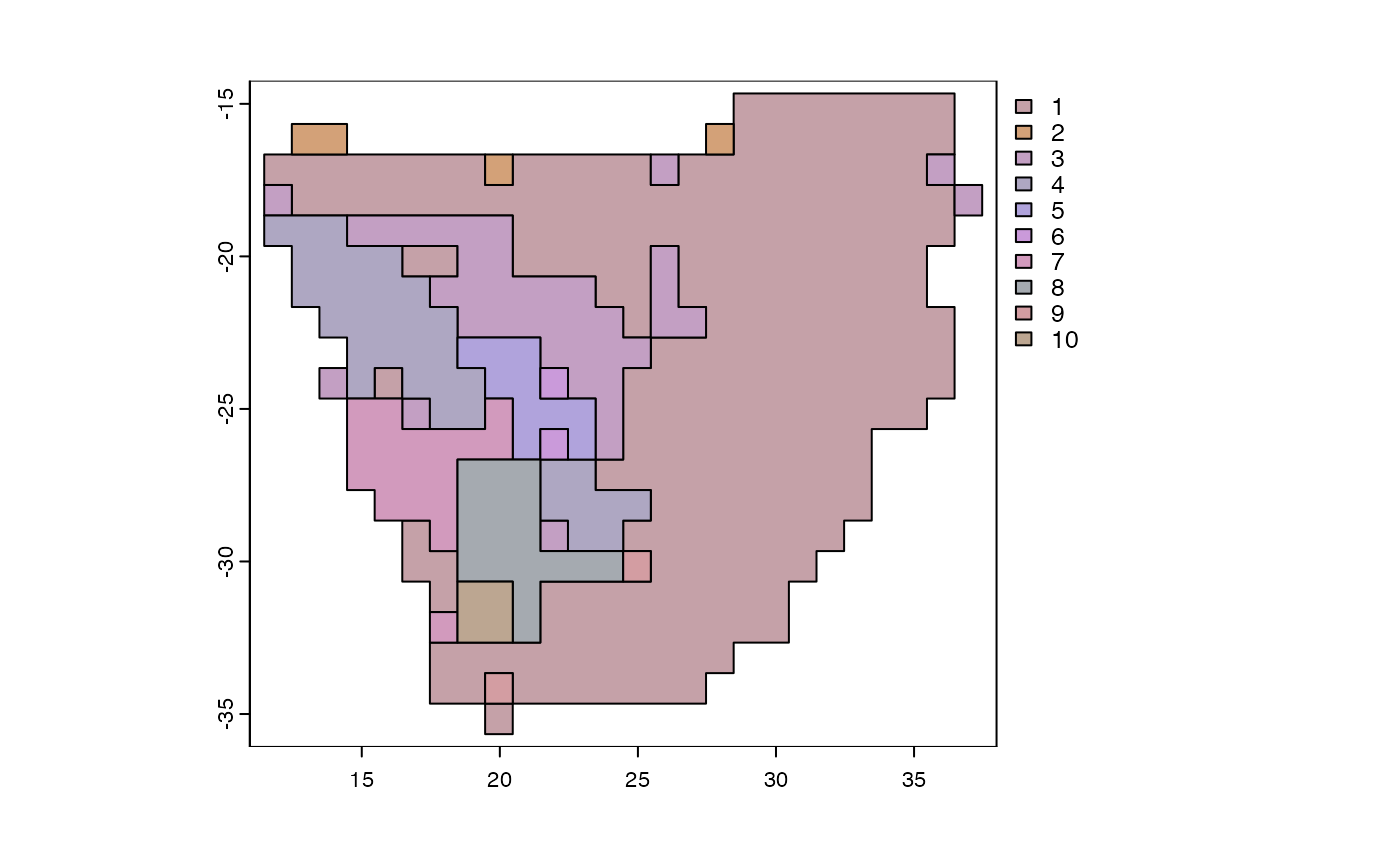Computes turnover of functional diversity using k-prototypes clustering algorithm tailored for mixed-type functional traits (numeric and categorical) to generate an integer vector of cluster assignments. The ranges of each species in a cluster are collapsed to generate a new community matrix based on the presence or absence of cluster membership in a grid cell. A grade of membership model or beta diversity is then fitted to the new reduced community matrix for further analysis.

functional_beta(
x,
trait = NULL,
bin = 10,
na.rm = FALSE,
quick_elbow = FALSE,
abundance = FALSE,
...
)

## Arguments

x

A dataframe or sparse community matrix of species occurrences.

trait

A data frame with the first column labeled “species” containing the taxonomic groups to be evaluated whereas the remaining columns contain the various functional traits. The variables should be mixed-type combining numeric and categorical variables.

bin

The desired number of clusters or bins. If elbow=TRUE, the optimal number of clusters is determined by running the analysis multiple times varying from 2 to bin.

na.rm

Logical, whether NA values should be removed prior to computation

quick_elbow

Quickly estimate the 'elbow' of a scree plot to determine the optimal number of clusters.

abundance

Logical, whether the reduced matrix should be returned as presence or absence of cluster representation or as abundances of cluster memberships

...

Further arguments passed to or from other methods.

## Value

A list with three dissimilarity matrices capturing: (i) turnover (replacement), (ii) nestedness-resultant component, and (iii) total dissimilarity (i.e. the sum of both components).

For index.family="sorensen" the three matrices are:

• beta.sim A distance object, dissimilarity matrix accounting for spatial turnover (replacement), measured as Simpson pair-wise dissimilarity.

• beta.sne dist object, dissimilarity matrix accounting for nestedness-resultant dissimilarity, measured as the nestedness-fraction of Sorensen pair-wise dissimilarity

• beta.sor dist object, dissimilarity matrix accounting for total dissimilarity, measured as Sorensen pair-wise dissimilarity (a monotonic transformation of beta diversity)

For index.family="jaccard" the three matrices are:

• beta.jtu A distance object, dissimilarity matrix accounting for spatial turnover, measured as the turnover-fraction of Jaccard pair-wise dissimilarity

• beta.jne dist object, dissimilarity matrix accounting for nestedness-resultant dissimilarity, measured as the nestedness-fraction of Jaccard pair-wise dissimilarity

• beta.jac dist object, dissimilarity matrix accounting for beta diversity, measured as Jaccard pair-wise dissimilarity (a monotonic transformation of beta diversity)

## Examples

# \donttest{
library(terra)
data(africa)
p <- vect(system.file("ex/sa.json", package = "phyloregion"))
fb <- functional_beta(x=africa$comm, trait = africa$trait)
#> Assuming range_size, habit, h_max, growth_duration, seed_size as functional traits.
#> # NAs in variables:
#>           habit growth_duration      range_size           h_max       seed_size
#>               6             340               0              18             686
#> Estimated lambda: 488220.6
#>
p <- phyloregion(fb[], pol = p)
#> Warning: convex combination of colors in polar coordinates (polarLUV) may not be appropriate
#> Warning: convex combination of colors in polar coordinates (polarLUV) may not be appropriate
plot(p)# }